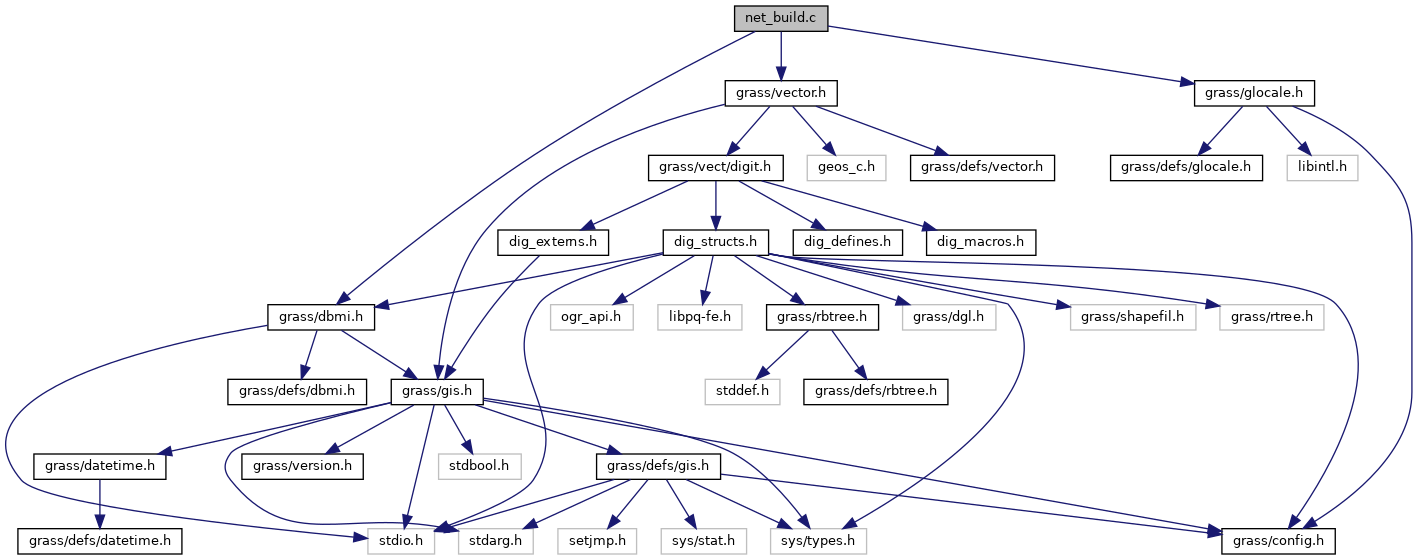GRASS GIS 7 Programmer's Manual  7.9.dev(2019)-f6edaaffa
net_build.c File Reference

Vector library - related fns for vector network building. More...

#include <grass/dbmi.h>
#include <grass/vector.h>
#include <grass/glocale.h>
Include dependency graph for net_build.c:Go to the source code of this file.

Functions

int Vect_net_ttb_build_graph (struct Map_info *Map, int ltype, int afield, int nfield, int tfield, int tucfield, const char *afcol, const char *abcol, const char *ncol, int geo, int algorithm)
Build network graph with turntable. More...

int Vect_net_build_graph (struct Map_info *Map, int ltype, int afield, int nfield, const char *afcol, const char *abcol, const char *ncol, int geo, int version)
Build network graph. More...

Detailed Description

Vector library - related fns for vector network building.

Higher level functions for reading/writing/manipulating vectors.

(C) 2001-2009, 2014 by the GRASS Development Team

This program is free software under the GNU General Public License (>=v2). Read the file COPYING that comes with GRASS for details.

Definition in file net_build.c.

◆ Vect_net_build_graph()

 int Vect_net_build_graph ( struct Map_info * Map, int ltype, int afield, int nfield, const char * afcol, const char * abcol, const char * ncol, int geo, int version )

Build network graph.

Internal format for edge costs is integer, costs are multiplied before conversion to int by 1000 and for lengths LL without geo flag by 1000000. The same multiplication factor is used for nodes. Costs in database column may be 'integer' or 'double precision' number >= 0 or -1 for infinity i.e. arc or node is closed and cannot be traversed If record in table is not found for arcs, arc is skip. If record in table is not found for node, costs for node are set to 0.

Parameters
 Map vector map ltype line type for arcs afield arc costs field (if 0, use length) nfield node costs field (if 0, do not use node costs) afcol column with forward costs for arc abcol column with backward costs for arc (if NULL, back costs = forward costs), ncol column with costs for nodes (if NULL, do not use node costs), geo use geodesic calculation for length (LL), version graph version to create (1, 2, 3)
Returns
0 on success, 1 on error

Definition at line 695 of file net_build.c.

◆ Vect_net_ttb_build_graph()

 int Vect_net_ttb_build_graph ( struct Map_info * Map, int ltype, int afield, int nfield, int tfield, int tucfield, const char * afcol, const char * abcol, const char * ncol, int geo, int algorithm )

Build network graph with turntable.

Internal format for edge costs is integer, costs are multiplied before conversion to int by 1000 and for lengths LL without geo flag by 1000000. The same multiplication factor is used for nodes. Costs in database column may be 'integer' or 'double precision' number >= 0 or -1 for infinity i.e. arc or node is closed and cannot be traversed If record in table is not found for arcs, costs for arc are set to 0. If record in table is not found for node, costs for node are set to 0.

Parameters
 Map vector map ltype line type for arcs afield arc costs field (if 0, use length) nfield node costs field (if 0, do not use node costs) tfield field where turntable is attached tucfield field with unique categories used in the turntable afcol column with forward costs for arc abcol column with backward costs for arc (if NULL, back costs = forward costs), ncol column with costs for nodes (if NULL, do not use node costs), geo use geodesic calculation for length (LL), algorithm not used (in future code for algorithm)
Returns
0 on success, 1 on error

Definition at line 48 of file net_build.c.